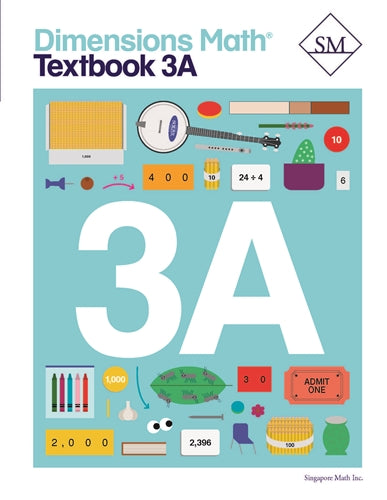# Dimensions Math Textbook 3A

Dimensions Math® Textbook 3A

Chapter 1: Numbers to 10,000

Lesson 1: Numbers to 10,000
Lesson 2: Place Value — Part 1
Lesson 3: Place Value — Part 2
Lesson 4: Comparing Numbers
Lesson 5: The Number Line
Lesson 6: Practice A
Lesson 7: Number Patterns
Lesson 8: Rounding to the Nearest Thousand
Lesson 9: Rounding to the Nearest Hundred
Lesson 10: Rounding to the Nearest Ten
Lesson 11: Practice B

Chapter 2: Addition and Subtraction — Part 1

Lesson 1: Mental Addition — Part 1
Lesson 2: Mental Addition — Part 2
Lesson 3: Mental Subtraction — Part 1
Lesson 4: Mental Subtraction — Part 2
Lesson 5: Making 100 and Making 1,000
Lesson 6: Strategies for Numbers Close to Hundreds
Lesson 7: Practice A
Lesson 8: Sum and Difference
Lesson 9: Word Problems — Part 1
Lesson 10: Word Problems — Part 2
Lesson 11: 2-Step Word Problems
Lesson 12: Practice B

Chapter 3: Addition and Subtraction — Part 2

Lesson 2: Subtraction with Regrouping — Part 1
Lesson 3: Subtraction with Regrouping — Part 2
Lesson 4: Estimating Sums and Differences — Part 1
Lesson 5: Estimating Sums and Differences — Part 2
Lesson 6: Word Problems
Lesson 7: Practice

Chapter 4: Multiplication and Division

Lesson 1: Looking Back at Multiplication
Lesson 2: Strategies for Finding the Product
Lesson 3: Looking Back at Division
Lesson 4: Multiplying and Dividing with 0 and 1
Lesson 5: Division with Remainders
Lesson 6: Odd and Even Numbers
Lesson 7: Word Problems — Part 1
Lesson 8: Word Problems — Part 2
Lesson 9: 2-Step Word Problems
Lesson 10: Practice
Review 1

Chapter 5: Multiplication

Lesson 1: Multiplying Ones, Tens, and Hundreds
Lesson 2: Multiplication Without Regrouping
Lesson 3: Multiplication with Regrouping Tens
Lesson 4: Multiplication with Regrouping Ones
Lesson 5: Multiplication with Regrouping Ones and Tens
Lesson 6: Practice A
Lesson 7: Multiply a 3-digit Number with Regrouping Once
Lesson 8: Multiplication with Regrouping More Than Once
Lesson 9: Practice B

Chapter 6: Division

Lesson 1: Dividing Tens and Hundreds
Lesson 2: Dividing a 2-digit Number by 2 — Part 1
Lesson 3: Dividing a 2-digit Number by 2 — Part 2
Lesson 4: Dividing a 2-digit Number by 3, 4, and 5
Lesson 5: Practice A
Lesson 6: Dividing a 3-digit Number by 2
Lesson 7: Dividing a 3-digit Number by 3, 4, and 5
Lesson 8: Dividing a 3-digit Number, Quotient is 2 Digits
Lesson 9: Practice B

Chapter 7: Graphs and Tables

Lesson 1: Picture Graphs and Bar Graphs
Lesson 2: Bar Graphs and Tables
Lesson 3: Practice
Review 2

Dimensions Math® PreK-5 series features the progression, rigor, and pacing that define Singapore math. Throughout the series, five characters offer students suggestions on how to think about problems. They remind students of strategies they’ve learned and point out important information that encourages them to come up with their own solutions.

Textbook lessons begin with a task that allows students to apply their previous knowledge and learn through discussion. Once students have mastered a concept with the use of concrete and pictorial aids, they are ready to take on more abstract mathematical problem sets. They reach fluency by collecting various strategies along the way and applying them to new problems. Word problems give students a sense of math in real-world contexts.

Workbooks offer independent practice that follows a careful progression of exercise variation. Each textbook lesson includes a corresponding workbook exercise that starts with pictorial representation and progresses to more challenging abstract problems. Workbooks for PreK-2 are perforated.

Teacher’s Guides include lesson plans, mathematical background, games, helpful suggestions, and comprehensive resources for daily lessons. Lessons are laid out clearly and activities are designed for the whole class, small groups, and extension.

Textbooks and Workbooks do not include answer keys. Answers are in Teacher's Guides.
Textbook:
ISBN  9781947226081